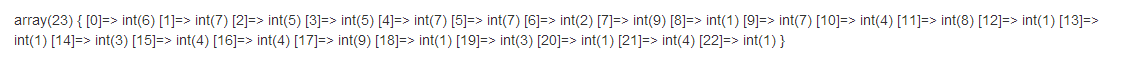# php随机数各种知识汇集

2018-04-25 16:18 更新

PHP的rand()函数将返回随机整数，具体使用方法如下：

``````rand(min,max)
``````

``echo(rand());?> ``## 使用PHP生成指定区间内的随机数

``````echo(rand(1000,2000));?>
``````

## 使用PHP获得一个集合中的随机元素

``````\$my_array=array('ASP','PHP','JAVASCRIPT','AJAX','CSS','JQUERY','HTML');
echo(\$my_array[rand(0,6)]);
?>
``````

``````\$my_array=array('ASP','PHP','JAVASCRIPT','AJAX','CSS','JQUERY','HTML');
\$repetition=rand(0,6);
for(\$i=0;\$i<=\$repetition;\$i++){
echo('I am learning ' . \$my_array[rand(0,6)]);
echo(' on w3cschool.cn');
}
?>
``````## PHP生成32位随机数

``````function genToken( \$len = 32, \$md5 = true ) {
# Seed random number generator
# Only needed for PHP versions prior to 4.2
mt_srand( (double)microtime()*1000000 );
# Array of characters, adjust as desired
\$chars = array(
'Q', '@', '8', 'y', '%', '^', '5', 'Z', '(', 'G', '_', 'O', '`',
'S', '-', 'N', '<', 'D', '{', '}', '[', ']', 'h', ';', 'W', '.',
'/', '|', ':', '1', 'E', 'L', '4', '&', '6', '7', '#', '9', 'a',
'A', 'b', 'B', '~', 'C', 'd', '>', 'e', '2', 'f', 'P', 'g', ')',
'?', 'H', 'i', 'X', 'U', 'J', 'k', 'r', 'l', '3', 't', 'M', 'n',
'=', 'o', '+', 'p', 'F', 'q', '!', 'K', 'R', 's', 'c', 'm', 'T',
'v', 'j', 'u', 'V', 'w', ',', 'x', 'I', '\$', 'Y', 'z', '*'
);
# Array indice friendly number of chars;
\$numChars = count(\$chars) - 1; \$token = '';
# Create random token at the specified length
for ( \$i=0; \$i<\$len; \$i++ )
\$token .= \$chars[ mt_rand(0, \$numChars) ];
# Should token be run through md5?
if ( \$md5 ) {
# Number of 32 char chunks
\$chunks = ceil( strlen(\$token) / 32 ); \$md5token = '';
# Run each chunk through md5
for ( \$i=1; \$i<=\$chunks; \$i++ )
\$md5token .= md5( substr(\$token, \$i * 32 - 32, 32) );
# Trim the token
\$token = substr(\$md5token, 0, \$len);
} return \$token;
}``````## php生成N个不重复的随机数

php生成N个不重复的随机数的方法：

``````<?php
/*
* array unique_rand（ int \$min, int \$max, int \$num ）
* 生成一定数量的不重复随机数
* \$min 和 \$max: 指定随机数的范围
* \$num: 指定生成数量
* site
*/
function unique_rand（\$min, \$max, \$num） {
\$count = 0;
\$return = array（）；
while （\$count < \$num） {
\$return[] = mt_rand（\$min, \$max）；
\$return = array_flip（array_flip（\$return））；
\$count = count（\$return）；
}
shuffle（\$return）；
return \$return;
}
\$arr = unique_rand（1, 25, 16）；
sort（\$arr）；
\$result = '';
for（\$i=0; \$i < count（\$arr）；\$i++）
{
\$result .= \$arr[\$i].',';
}
\$result = substr（\$result, 0, -1）；
echo \$result;
?>
运行结果：
2,3,4,6,7,8,9,10,11,12,13,16,20,21,22,24
``````

## 用php成n个随机数，要求n个数的和等于100

``````\$rand_array = array();
function  get_rand_n(\$rand_array) {
\$rand_number = mt_rand(1,9);
if(empty(\$rand_array)) {
\$rand_array[] = \$rand_number;
return get_rand_n(\$rand_array);
} else {
\$count = 0;
foreach(\$rand_array as \$item) {
\$count += \$item;
}
if(\$count<100) {
if(\$count+\$rand_number == 100) {
\$rand_array[] = \$rand_number;
return \$rand_array;
} else if(\$count+\$rand_number < 100) {
\$rand_array[] = \$rand_number;
return get_rand_n(\$rand_array); // 回掉再次计算
} else { // 如果得到的值大于了100
return get_rand_n(\$rand_array); // 重新获得随机数，知道为100的时候返回这个随机数数组
}
}
}
}
\$rand_array = get_rand_n(\$rand_array);
var_dump(\$rand_array);
``````## php获取四位字母和数字的随机数

``````<?php
function GetfourStr(\$len)
{
\$chars_array = array(
"0", "1", "2", "3", "4", "5", "6", "7", "8", "9",
"a", "b", "c", "d", "e", "f", "g", "h", "i", "j", "k",
"l", "m", "n", "o", "p", "q", "r", "s", "t", "u", "v",
"w", "x", "y", "z", "A", "B", "C", "D", "E", "F", "G",
"H", "I", "J", "K", "L", "M", "N", "O", "P", "Q", "R",
"S", "T", "U", "V", "W", "X", "Y", "Z",
);
\$charsLen = count(\$chars_array) - 1;

\$outputstr = "";
for (\$i=0; \$i<\$len; \$i++)
{
\$outputstr .= \$chars_array[mt_rand(0, \$charsLen)];
}
return \$outputstr;
}
echo GetfourStr(4);
?>

``````## php生成指定长度的随机数

``````<?php
function get_random(\$len=3){
//range 是将10到99列成一个数组
\$numbers = range (10,99);
//shuffle 将数组顺序随即打乱
shuffle (\$numbers);
//取值起始位置随机
\$start = mt_rand(1,10);
//取从指定定位置开始的若干数
\$result = array_slice(\$numbers,\$start,\$len);
\$random = "";
for (\$i=0;\$i<\$len;\$i++){
\$random = \$random.\$result[\$i];
}
return \$random;
}

//随机数
function get_random2(\$length = 4) {
\$min = pow(10 , (\$length - 1));
\$max = pow(10, \$length) - 1;
return mt_rand(\$min, \$max);
}

echo "<br/>";
echo get_random(3);
echo "<br/>";
echo get_random2(6);
``````

## php生成随机密码的三种方法

1、在 33 – 126 中生成一个随机整数，如 35，

2、将 35 转换成对应的ASCII码字符 #

3、重复以上 1、2 步骤 n 次，连接成 n 位的密码

``````function create_password(\$pw_length = 8)
{
\$randpwd = '';
for (\$i = 0; \$i < \$pw_length; \$i++)
{
\$randpwd .= chr(mt_rand(33, 126));
}
return \$randpwd;
}
// 调用该函数，传递长度参数\$pw_length = 6
``````

1、预置一个的字符串 \$chars ，包括 a – z，A – Z，0 – 9，以及一些特殊字符

2、在 \$chars 字符串中随机取一个字符

3、重复第二步 n 次，可得长度为 n 的密码
``````function generate_password( \$length = 8 ) {
// 密码字符集，可任意添加你需要的字符
\$chars = 'abcdefghijklmnopqrstuvwxyzABCDEFGHIJKLMNOPQRSTUVWXYZ0123456789!@#\$%^&*()-_ []{}<>~`+=,.;:/?|';
for ( \$i = 0; \$i < \$length; \$i++ )
{
// 这里提供两种字符获取方式
// 第一种是使用 substr 截取\$chars中的任意一位字符；
// 第二种是取字符数组 \$chars 的任意元素
// \$password .= substr(\$chars, mt_rand(0, strlen(\$chars) - 1), 1);
\$password .= \$chars[ mt_rand(0, strlen(\$chars) - 1) ];
}
}
``````

1、预置一个的字符数组 \$chars ，包括 a – z，A – Z，0 – 9，以及一些特殊字符

2、通过array_rand()从数组 \$chars 中随机选出 \$length 个元素

3、根据已获取的键名数组 \$keys，从数组 \$chars 取出字符拼接字符串。该方法的缺点是相同的字符不会重复取。
``````function make_password( \$length = 8 )
{
// 密码字符集，可任意添加你需要的字符
\$chars = array('a', 'b', 'c', 'd', 'e', 'f', 'g', 'h',
'i', 'j', 'k', 'l','m', 'n', 'o', 'p', 'q', 'r', 's',
't', 'u', 'v', 'w', 'x', 'y','z', 'A', 'B', 'C', 'D',
'E', 'F', 'G', 'H', 'I', 'J', 'K', 'L','M', 'N', 'O',
'P', 'Q', 'R', 'S', 'T', 'U', 'V', 'W', 'X', 'Y','Z',
'0', '1', '2', '3', '4', '5', '6', '7', '8', '9', '!',
'@','#', '\$', '%', '^', '&', '*', '(', ')', '-', '_',
'[', ']', '{', '}', '<', '>', '~', '`', '+', '=', ',',
'.', ';', ':', '/', '?', '|');
// 在 \$chars 中随机取 \$length 个数组元素键名
\$keys = (\$chars, \$length);
for(\$i = 0; \$i < \$length; \$i++)
{
// 将 \$length 个数组元素连接成字符串
}
}
``````

``````<?php
function getmicrotime()
{
list(\$usec, \$sec) = explode(" ",microtime());
return ((float)\$usec + (float)\$sec);
}
// 记录开始时间
\$time_start = getmicrotime();
// 这里放要执行的PHP代码，如:
// 记录结束时间
\$time_end = getmicrotime();
\$time = \$time_end - \$time_start;
// 输出运行总时间
echo "执行时间 \$time seconds";
?>
``````

App下载